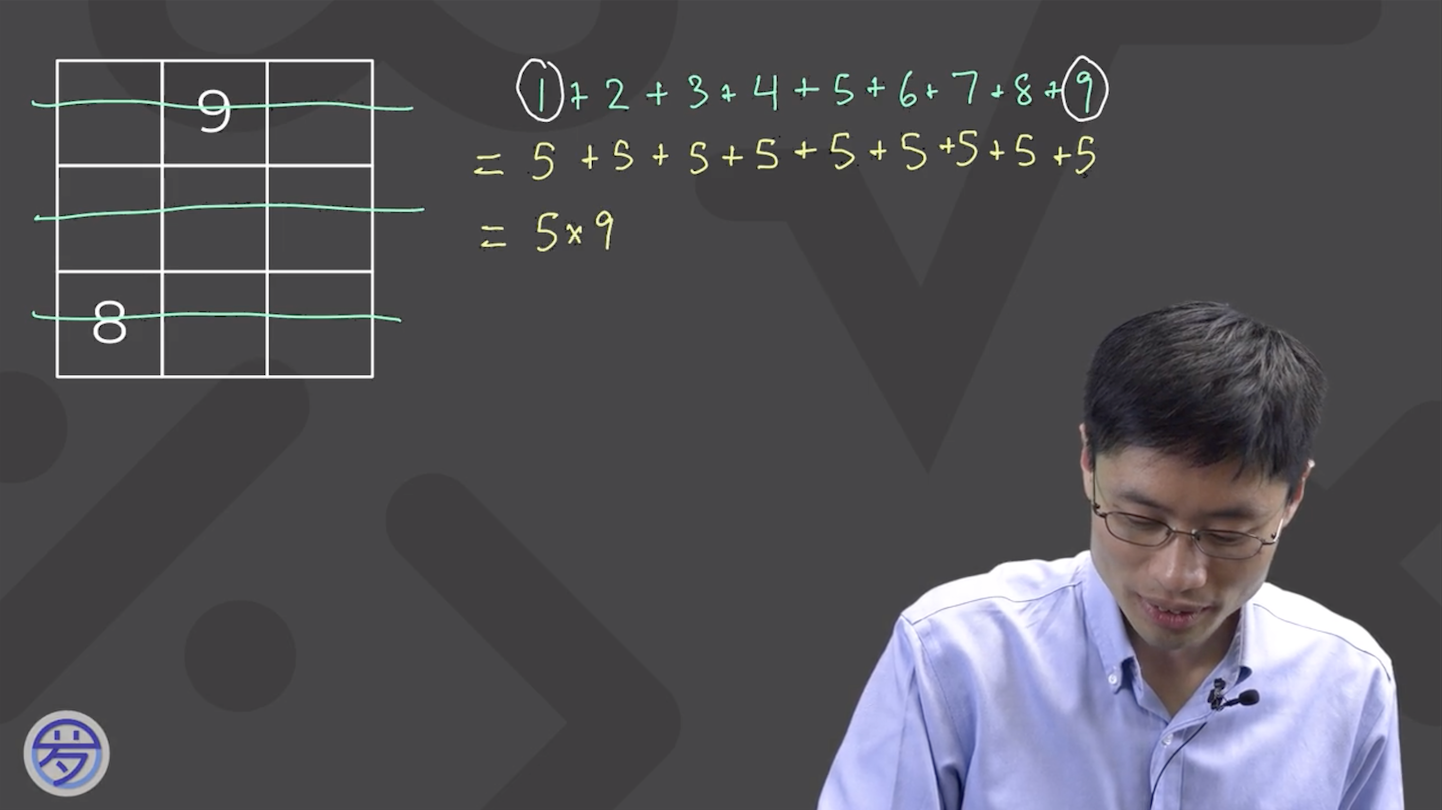# Middle number

• In a magic square, is the middle number always the average of all the other numbers? It seemed to be the pattern in all the magic squares in this module.

• Hello!

That is an excellent observation! You're correct, except it only applies for $$n \times n$$ squares where $$n =$$ odd number ($$3, 5, 7,$$ etc.). This is because for squares with even number of rows and columns, there is no "middle" number.

To generalize for all squares with an odd number of rows/columns, I'm going to refer to the Challenge Explanation 1 of 3 video as shown below:The above screenshot shows the sum of all numbers in a 3x3 magic square. Similarly, In an $$n \times n$$ square:
Sum $$= 1+2+3+...+n^2$$
$$= \frac{(n^2+1)}{2} + \frac{(n^2+1)}{2} + \frac{(n^2+1)}{2}+...+\frac{(n^2+1)}{2}$$ [$$n^2$$ times]
$$= \frac{(n^2+1)}{2} \times n^2$$

Since there are $$n^2$$ numbers, the average of all numbers in the square is:
$$[\frac{(n^2+1)}{2} \times n^2] \div n^2 = \frac{(n^2+1)}{2}$$

Let's check: For a 3x3 square, Prof. Loh showed that the center number is 5. Plugging in 3 for n, $$\frac{(n^2+1)}{2} = \frac{(3^2+1)}{2} = 5$$.
Therefore, for an $$n \times n$$ square, the center number will always be $$\frac{(n^2+1)}{2}$$.

Hope this helps!

Happy Learning,
The Daily Challenge Team

• Hi Minji

Thanks for the answer. I am still a bit confused. Haven't you just shown that the average of the sum of all the numbers equals n squared plus one all over 2? How does this prove that the middle number of the magic square is always the average?

Thank you

• Hi again!

I apologize for the confusion. I had misunderstood your question and tried to show how to calculate the average of all numbers in the square. The middle number is not necessarily the average of all the other numbers. Great observation, though!

Happy Learning,
The Daily Challenge Team

• Hi @ingeniousosprey See, there's another way to do it. In the first magic square the numbers were 1, 2, 3, 4, 5, 6, 7, 8, 9. The median is 5 in this list. That is the middle number in the first magic square. The sum will be the median of the list times 3. It works for the second magic square too.

• Also, yes. The mean is all the numbers sum divided how much numbers there are.